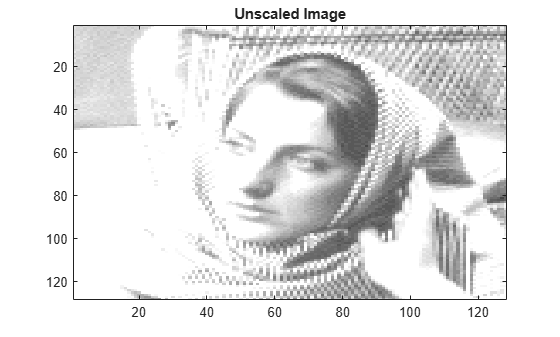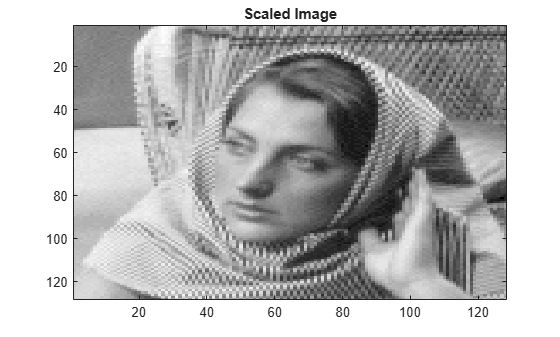# wcodemat

Extended pseudocolor matrix scaling

## Syntax

```Y = wcodemat(X) Y = wcodemat(X,NBCODES) Y = wcodemat(X,NBCODES,OPT) Y = wcodemat(X,NBCODES,OPT,ABSOL) ```

## Description

`wcodemat` rescales an input matrix to a specified range for display. If the specified range is the full range of the current colormap, `wcodemat` is similar in behavior to `imagesc`.

`Y = wcodemat(X)` rescales the matrix `X` to integers in the range [1,16].

`Y = wcodemat(X,NBCODES)` rescales the input `X` as integers in the range [1,`NBCODES`] . The default value of `NBCODES` is 16.

`Y = wcodemat(X,NBCODES,OPT)` rescales the matrix along the dimension specified by `OPT`. `OPT` can be one of: `'column'` (or `'c'`), `'row'` (or `'r'`), and `'mat'` (or `'m'`). `'rows'` scales `X` row-wise, `'column'` scales `X` column-wise, and `'mat'` scales `X` globally. The default value of `OPT` is `'mat'`.

`Y = wcodemat(X,NBCODES,OPT,ABSOL)` rescales the input matrix `X` based on the absolute values of the entries in `X` if `ABSOL` is nonzero, or based on the signed values of `X` if `ABSOL` is equal to zero. The default value of `ABSOL` is 1.

## Examples

collapse all

Scale level-one approximation coefficients globally to the full range of the colormap.

`load woman;`

Get the range of the colormap.

`NBCOL = size(map,1);`

Obtain the 2D dwt using the Haar wavelet.

`[cA1,cH1,cV1,cD1] = dwt2(X,'db1');`

Display without scaling and with scaling.

```image(cA1); colormap(map); title('Unscaled Image');``````figure image(wcodemat(cA1,NBCOL)); colormap(map); title('Scaled Image');```#### Introduced before R2006a

##### SupportGet trial now